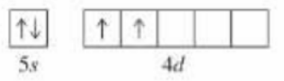# Problem: Identify the element which has the following partial orbital diagram a. Ti b. Sn c. Ge d. Zr e. Pr

###### FREE Expert Solution
85% (461 ratings)
###### Problem Details

Identify the element which has the following partial orbital diagram

a. Ti

b. Sn

c. Ge

d. Zr

e. PrFrequently Asked Questions

What scientific concept do you need to know in order to solve this problem?

Our tutors have indicated that to solve this problem you will need to apply the The Electron Configuration concept. You can view video lessons to learn The Electron Configuration. Or if you need more The Electron Configuration practice, you can also practice The Electron Configuration practice problems.

What is the difficulty of this problem?

Our tutors rated the difficulty ofIdentify the element which has the following partial orbital...as low difficulty.

How long does this problem take to solve?

Our expert Chemistry tutor, Jules took 1 minute and 47 seconds to solve this problem. You can follow their steps in the video explanation above.

What professor is this problem relevant for?

Based on our data, we think this problem is relevant for Professor Randles' class at UCF.